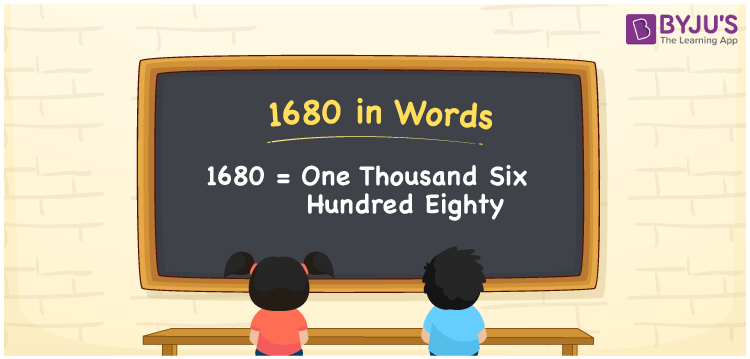# 1680 in words

1680 in words is written as One Thousand Six Hundred and Eighty. 1680 represents the count or value. The article on Counting Numbers can give you an idea about count or counting. The number 1680 is used in expressions that relate to money, distance, length, year and others. Let us consider an example for 1680. “By the year One Thousand Six Hundred and Eighty, Portugal, French, Dutch and English had all invaded India”. Another example, “All the Thousand Six Hundred and Eighty Parking spaces at First floor in a Mall were occupied.”

 1680 in words One Thousand Six Hundred and Eighty One Thousand Six Hundred and Eighty in Numbers 1680

## 1680 in English Words## How to Write 1680 in Words?

We can convert 1680 to words using a place value chart. The number 1680 has 4 digits, so let’s make a chart that shows the place value up to 4 digits.

 Thousands Hundreds Tens Ones 1 6 8 0

Thus, we can write the expanded form as:

1 × Thousand + 6 × Hundred + 8 × Ten + 0 × One

= 1 × 1000 + 6 × 100 + 8 × 10 + 0 × 1

= 1680

= One Thousand Six Hundred and Eighty.

1680 is the natural number that is succeeded by 1679 and preceded by 1681.

1680 in words – One Thousand Six Hundred and Eighty.

Is 1680 an odd number? – No.

Is 1680 an even number? – Yes.

Is 1680 a perfect square number? – No.

Is 1680 a perfect cube number? – No.

Is 1680 a prime number? – No.

Is 1680 a composite number? – Yes.

## Solved Example

1. Write the number 1680 in expanded form

Solution: 1 × 1000 + 6 × 100 + 8 × 10 + 0 × 1.

We can write 1680 = 1000 + 600 + 80 + 0

= 1 × 1000 + 6 × 100 + 8 × 10 + 0 × 1.

## Frequently Asked Questions on 1680 in words

Q1

### How to write 1680 in words?

1680 in words is written as One Thousand Six Hundred and Eighty.
Q2

### State whether True or False. 1680 is divisible by 3?

True. 1680 is divisible by 3.
Q3

### Is 1680 divisible by 10?

Yes. 1680 is divisible by 10.